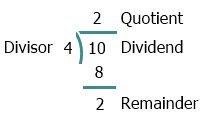# Java Program to Find Quotient and Remainder

Before creating the program, let's remind all the terms such as divisor, quotient, dividend, and remainder using the following figure:Here, the dividend can also be referred to as the numerator, and the divisor can also be referred to as the denominator.

## Find Quotient and Remainder in Java

The question is: write a Java program to find and print the quotient and the remainder value. The data say dividend and divisor must be received by the user at runtime. The program given below is an answer to this question:

```import java.util.Scanner;

public class CodesCracker
{
public static void main(String[] args)
{
float numerator, denominator;
int quotient, remainder;
Scanner s = new Scanner(System.in);

System.out.print("Enter the Dividend: ");
numerator = s.nextFloat();
System.out.print("Enter the Divisor: ");
denominator = s.nextFloat();

quotient = (int) (numerator/denominator);
remainder = (int) (numerator%denominator);

System.out.println("\nQuotient = " +quotient);
System.out.println("Remainder = " +remainder);
}
}```

Here is its sample run with user input: 21 as dividend and 8 as divisor:When dividing the number 21 by 8, we will get 2 as the quotient and 5 as the remainder. That is, 8 (divisor) * 2 (quotient) + 5 (remainder) = 21 (dividend).

The program begins by declaring two float variables, numerator and denominator, as well as two integer variables, quotient and remainder. It also declares the Scanner object s.

The program then prompts the user to input the dividend and divisor using the Scanner object's nextFloat() method and assigns these values to the numerator and denominator variables, respectively.

The program then calculates the quotient and remainder of the division of these two numbers using the % and / operators and assigns the results to the quotient and remainder variables, respectively. The int casting is used to ensure that the result of the division is an integer.

The program then prints out the values of the quotient and remainder variables using the System.out.println() method.

Java Online Test

« Previous Program Next Program »